Скачать презентацию 5 -2 Bisectors in Triangles Warm Up Lesson

6617388514360b6d4e999ddd5459b569.ppt

• Количество слайдов: 30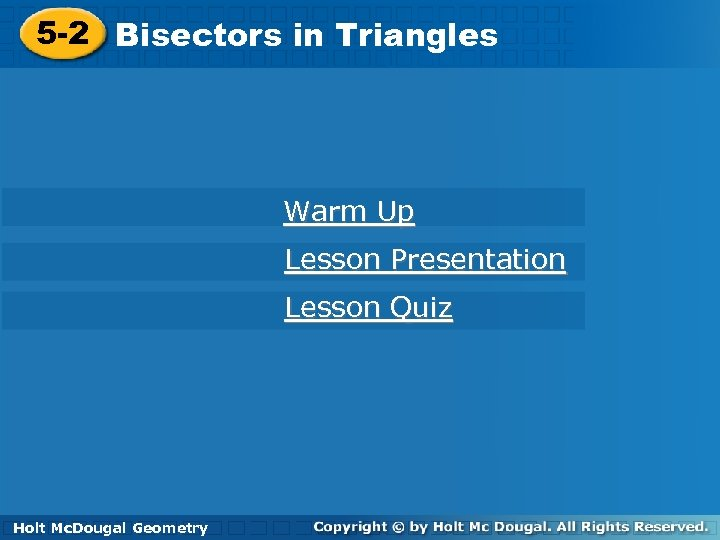5 -2 Bisectors in Triangles Warm Up Lesson Presentation Lesson Quiz Holt Geometry Holt Mc. Dougal Geometry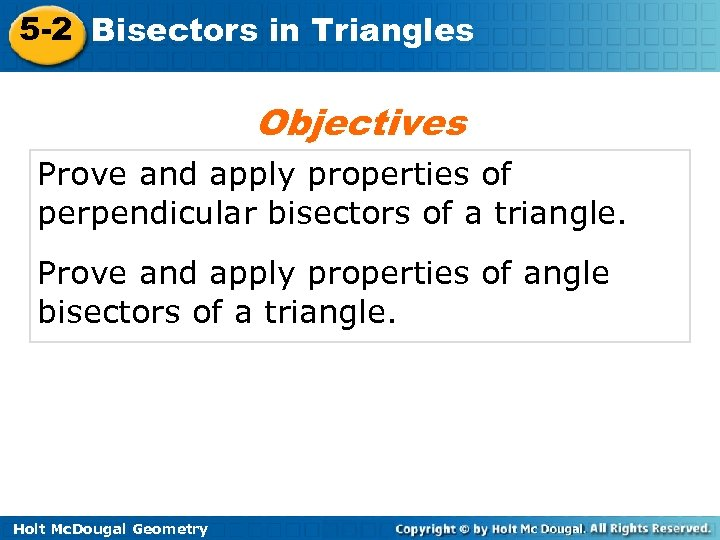5 -2 Bisectors in Triangles Objectives Prove and apply properties of perpendicular bisectors of a triangle. Prove and apply properties of angle bisectors of a triangle. Holt Mc. Dougal Geometry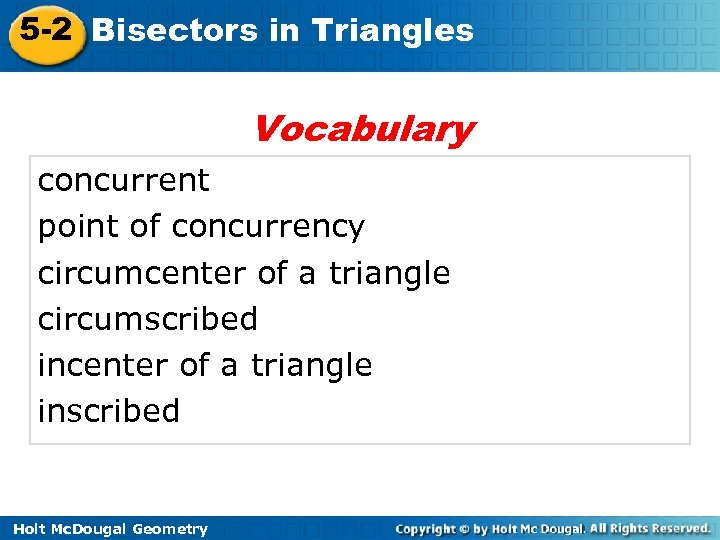5 -2 Bisectors in Triangles Vocabulary concurrent point of concurrency circumcenter of a triangle circumscribed incenter of a triangle inscribed Holt Mc. Dougal Geometry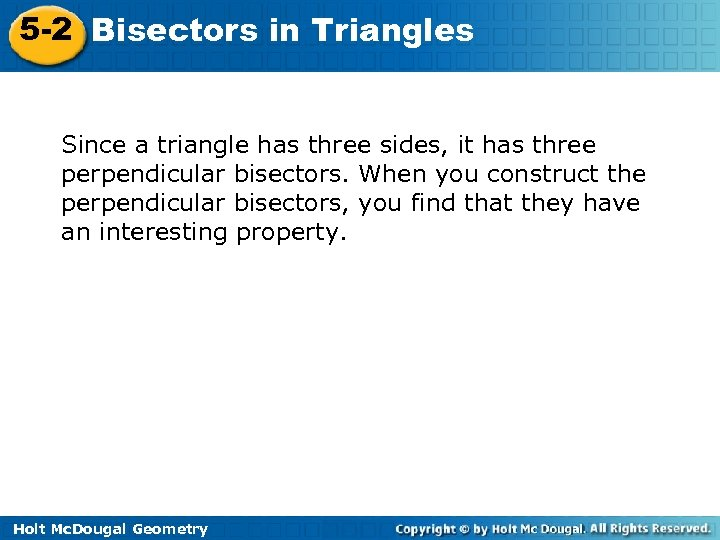5 -2 Bisectors in Triangles Since a triangle has three sides, it has three perpendicular bisectors. When you construct the perpendicular bisectors, you find that they have an interesting property. Holt Mc. Dougal Geometry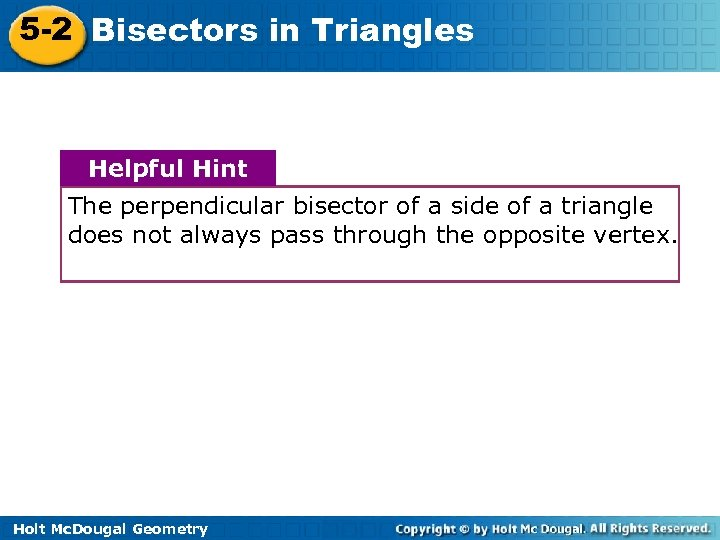5 -2 Bisectors in Triangles Helpful Hint The perpendicular bisector of a side of a triangle does not always pass through the opposite vertex. Holt Mc. Dougal Geometry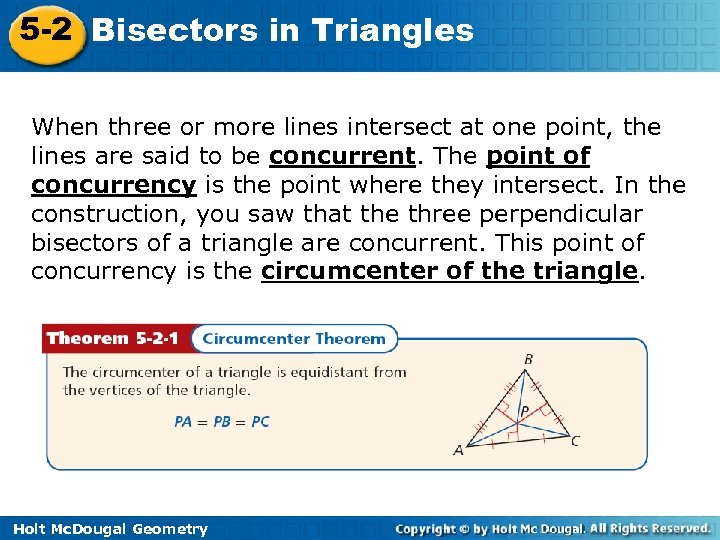5 -2 Bisectors in Triangles When three or more lines intersect at one point, the lines are said to be concurrent. The point of concurrency is the point where they intersect. In the construction, you saw that the three perpendicular bisectors of a triangle are concurrent. This point of concurrency is the circumcenter of the triangle. Holt Mc. Dougal Geometry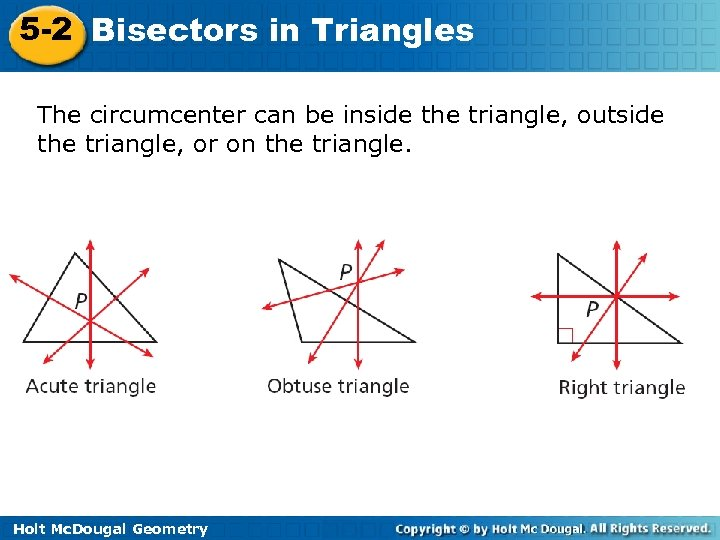5 -2 Bisectors in Triangles The circumcenter can be inside the triangle, outside the triangle, or on the triangle. Holt Mc. Dougal Geometry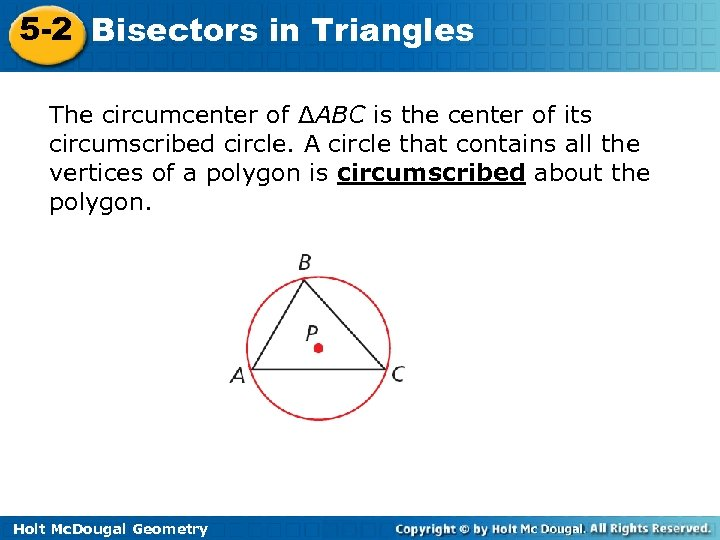5 -2 Bisectors in Triangles The circumcenter of ΔABC is the center of its circumscribed circle. A circle that contains all the vertices of a polygon is circumscribed about the polygon. Holt Mc. Dougal Geometry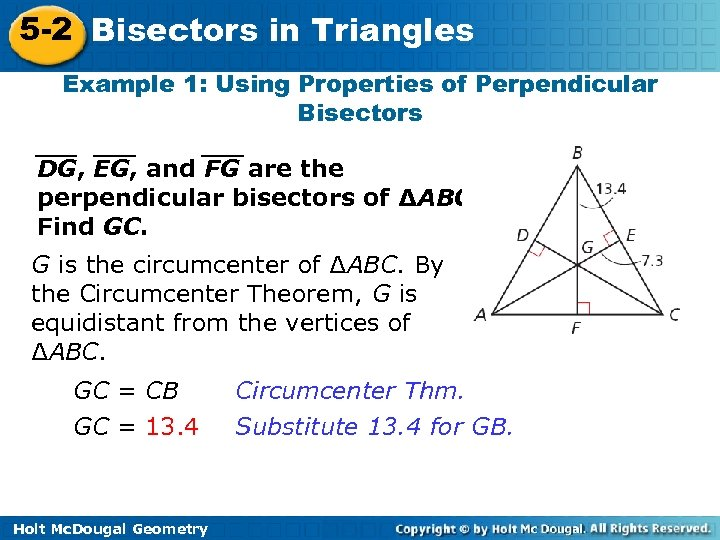5 -2 Bisectors in Triangles Example 1: Using Properties of Perpendicular Bisectors DG, EG, and FG are the perpendicular bisectors of ∆ABC. Find GC. G is the circumcenter of ∆ABC. By the Circumcenter Theorem, G is equidistant from the vertices of ∆ABC. GC = CB GC = 13. 4 Holt Mc. Dougal Geometry Circumcenter Thm. Substitute 13. 4 for GB.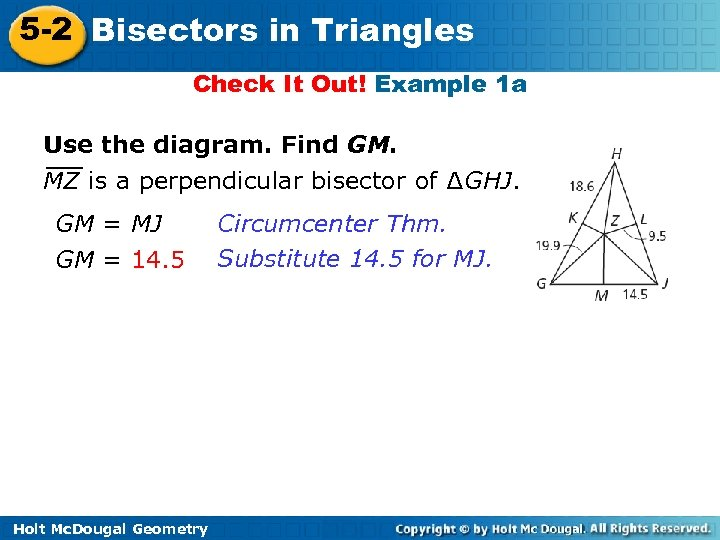5 -2 Bisectors in Triangles Check It Out! Example 1 a Use the diagram. Find GM. MZ is a perpendicular bisector of ∆GHJ. GM = MJ GM = 14. 5 Holt Mc. Dougal Geometry Circumcenter Thm. Substitute 14. 5 for MJ.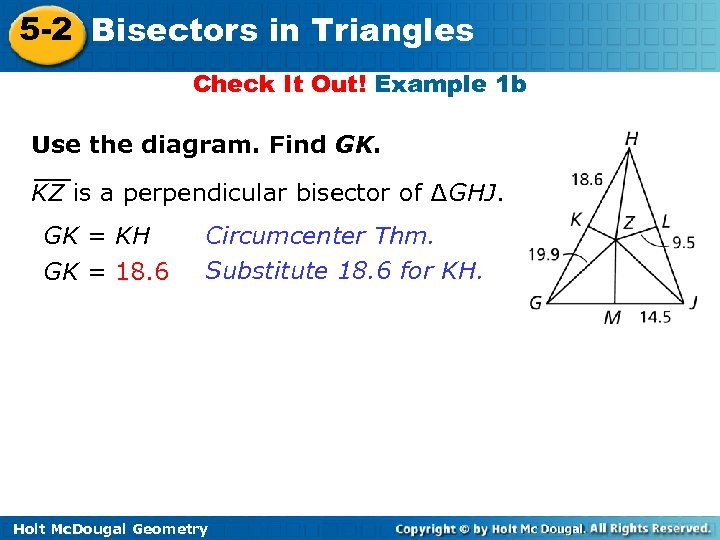5 -2 Bisectors in Triangles Check It Out! Example 1 b Use the diagram. Find GK. KZ is a perpendicular bisector of ∆GHJ. GK = KH GK = 18. 6 Circumcenter Thm. Substitute 18. 6 for KH. Holt Mc. Dougal Geometry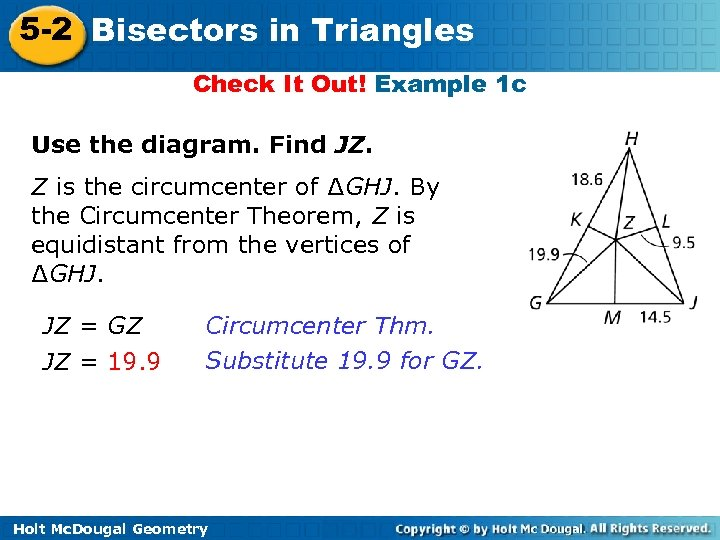5 -2 Bisectors in Triangles Check It Out! Example 1 c Use the diagram. Find JZ. Z is the circumcenter of ∆GHJ. By the Circumcenter Theorem, Z is equidistant from the vertices of ∆GHJ. JZ = GZ JZ = 19. 9 Circumcenter Thm. Substitute 19. 9 for GZ. Holt Mc. Dougal Geometry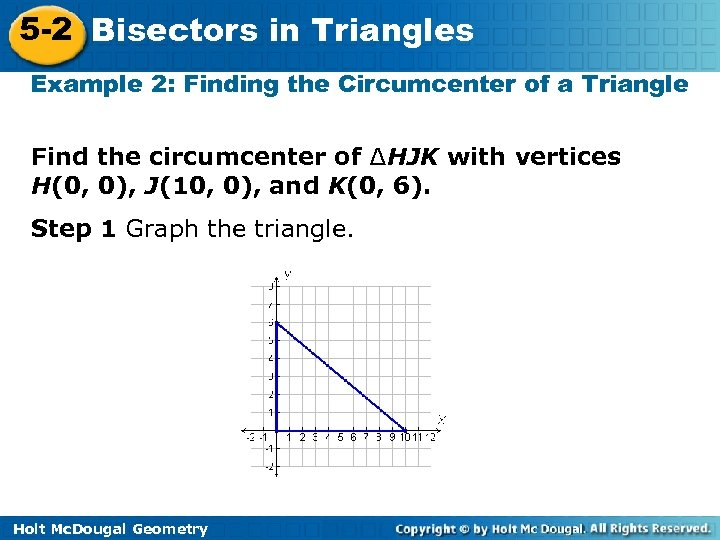5 -2 Bisectors in Triangles Example 2: Finding the Circumcenter of a Triangle Find the circumcenter of ∆HJK with vertices H(0, 0), J(10, 0), and K(0, 6). Step 1 Graph the triangle. Holt Mc. Dougal Geometry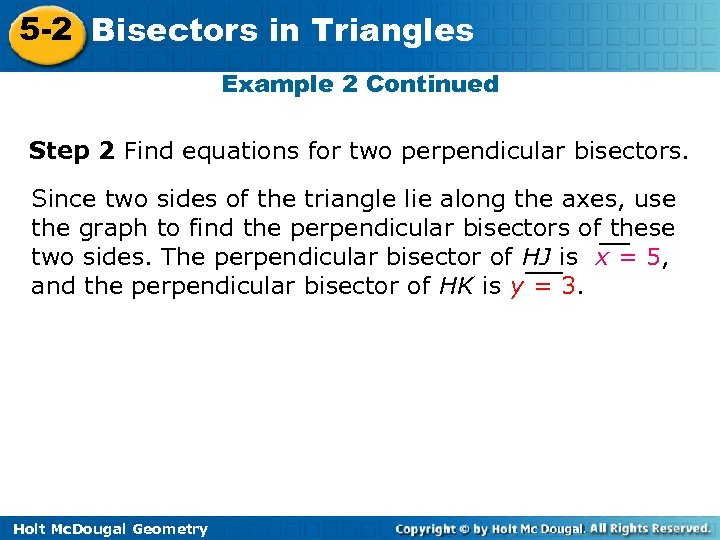5 -2 Bisectors in Triangles Example 2 Continued Step 2 Find equations for two perpendicular bisectors. Since two sides of the triangle lie along the axes, use the graph to find the perpendicular bisectors of these two sides. The perpendicular bisector of HJ is x = 5, and the perpendicular bisector of HK is y = 3. Holt Mc. Dougal Geometry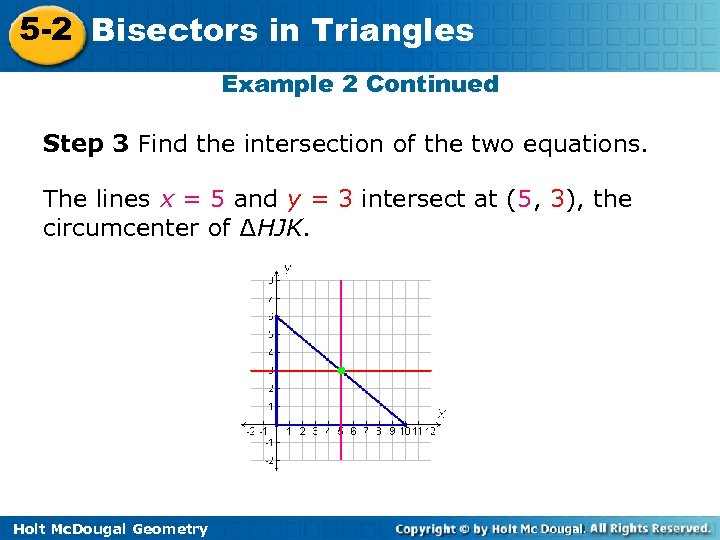5 -2 Bisectors in Triangles Example 2 Continued Step 3 Find the intersection of the two equations. The lines x = 5 and y = 3 intersect at (5, 3), the circumcenter of ∆HJK. Holt Mc. Dougal Geometry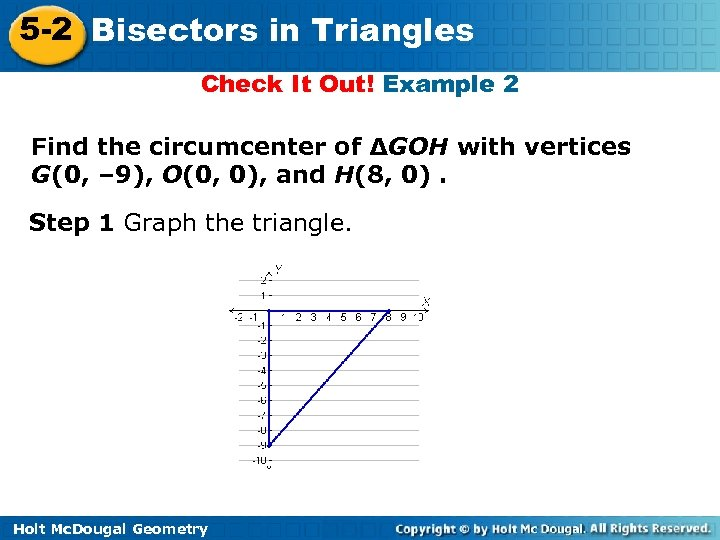5 -2 Bisectors in Triangles Check It Out! Example 2 Find the circumcenter of ∆GOH with vertices G(0, – 9), O(0, 0), and H(8, 0). Step 1 Graph the triangle. Holt Mc. Dougal Geometry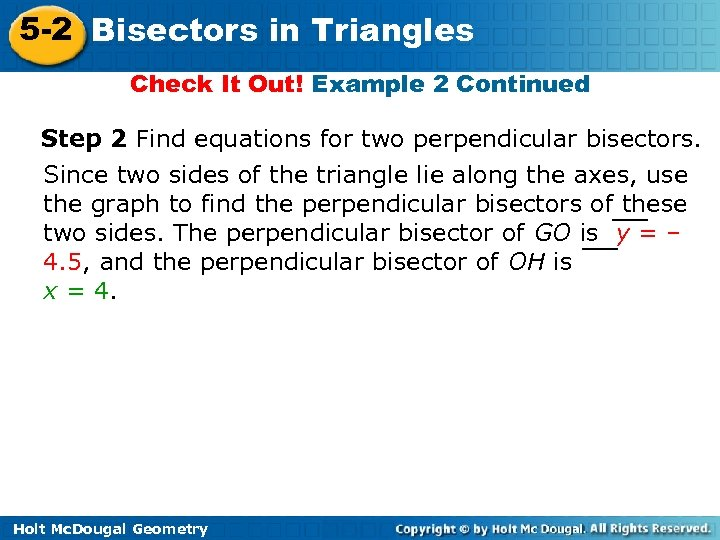5 -2 Bisectors in Triangles Check It Out! Example 2 Continued Step 2 Find equations for two perpendicular bisectors. Since two sides of the triangle lie along the axes, use the graph to find the perpendicular bisectors of these two sides. The perpendicular bisector of GO is y = – 4. 5, and the perpendicular bisector of OH is x = 4. Holt Mc. Dougal Geometry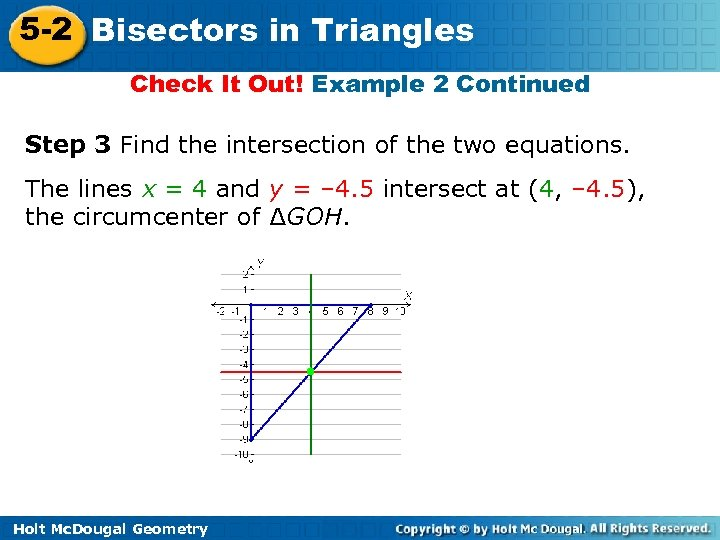5 -2 Bisectors in Triangles Check It Out! Example 2 Continued Step 3 Find the intersection of the two equations. The lines x = 4 and y = – 4. 5 intersect at (4, – 4. 5), the circumcenter of ∆GOH. Holt Mc. Dougal Geometry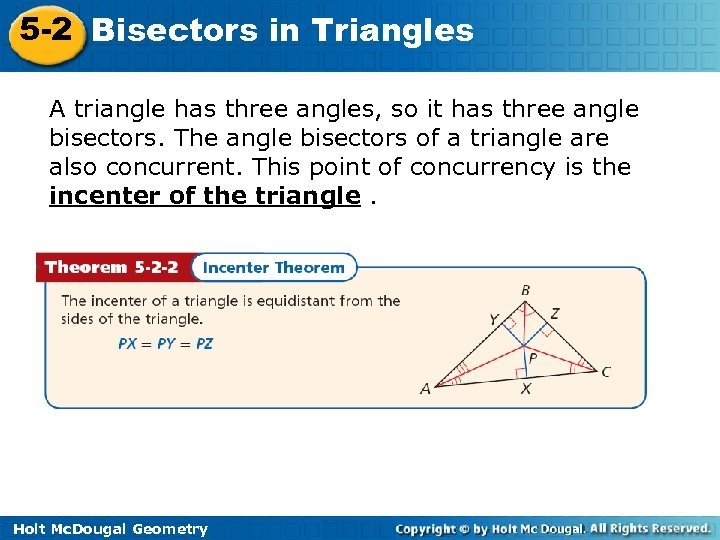5 -2 Bisectors in Triangles A triangle has three angles, so it has three angle bisectors. The angle bisectors of a triangle are also concurrent. This point of concurrency is the incenter of the triangle. Holt Mc. Dougal Geometry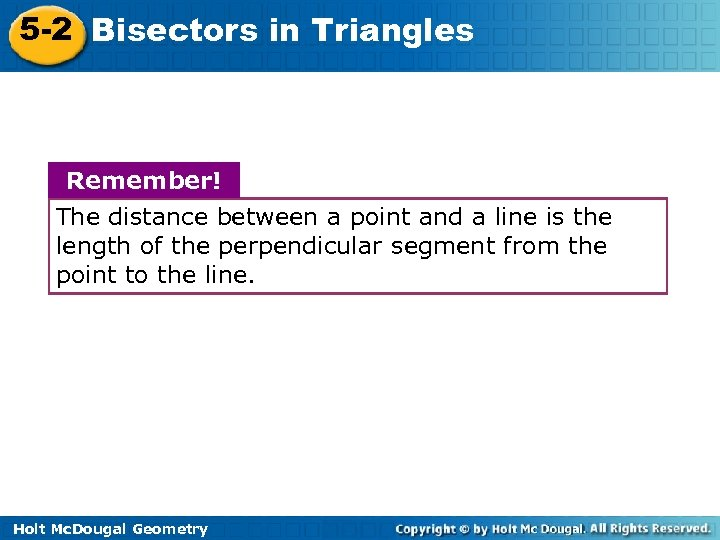5 -2 Bisectors in Triangles Remember! The distance between a point and a line is the length of the perpendicular segment from the point to the line. Holt Mc. Dougal Geometry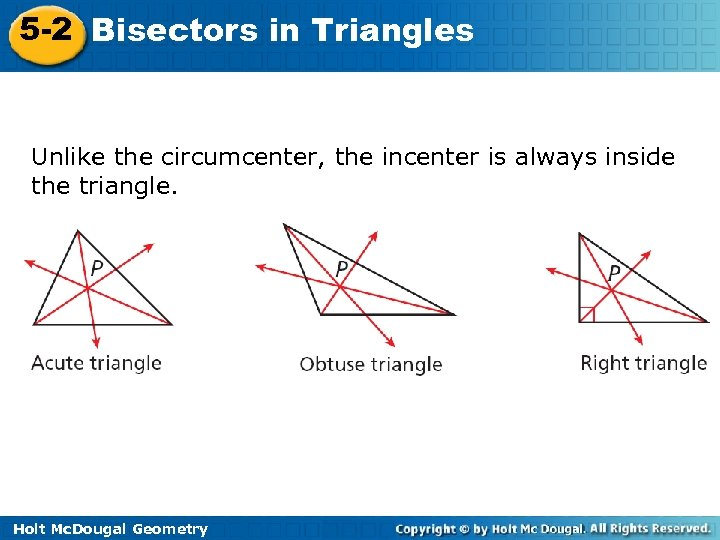5 -2 Bisectors in Triangles Unlike the circumcenter, the incenter is always inside the triangle. Holt Mc. Dougal Geometry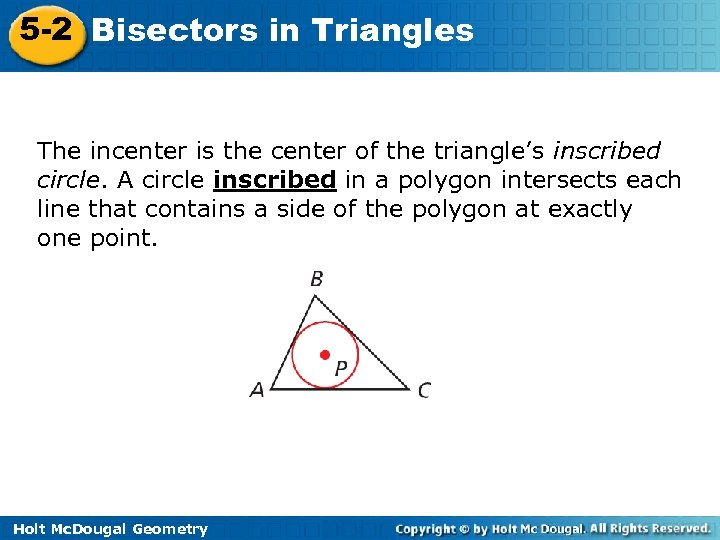5 -2 Bisectors in Triangles The incenter is the center of the triangle’s inscribed circle. A circle inscribed in a polygon intersects each line that contains a side of the polygon at exactly one point. Holt Mc. Dougal Geometry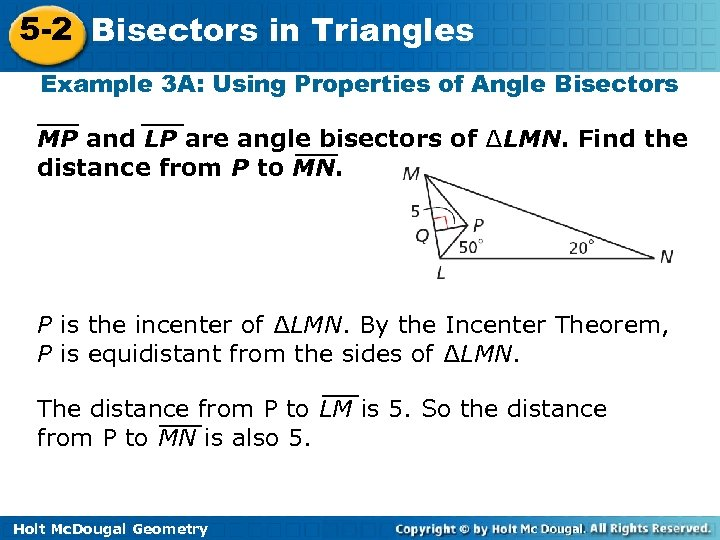5 -2 Bisectors in Triangles Example 3 A: Using Properties of Angle Bisectors MP and LP are angle bisectors of ∆LMN. Find the distance from P to MN. P is the incenter of ∆LMN. By the Incenter Theorem, P is equidistant from the sides of ∆LMN. The distance from P to LM is 5. So the distance from P to MN is also 5. Holt Mc. Dougal Geometry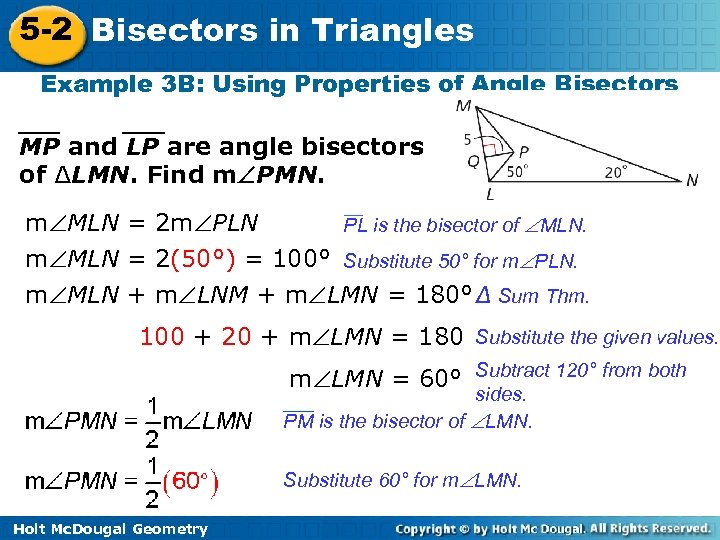5 -2 Bisectors in Triangles Example 3 B: Using Properties of Angle Bisectors MP and LP are angle bisectors of ∆LMN. Find m PMN. m MLN = 2 m PLN PL is the bisector of MLN. m MLN = 2(50°) = 100° Substitute 50° for m PLN. m MLN + m LNM + m LMN = 180° Δ Sum Thm. 100 + 20 + m LMN = 180 Substitute the given values. m LMN = 60° Subtract 120° from both sides. PM is the bisector of LMN. Substitute 60° for m LMN. Holt Mc. Dougal Geometry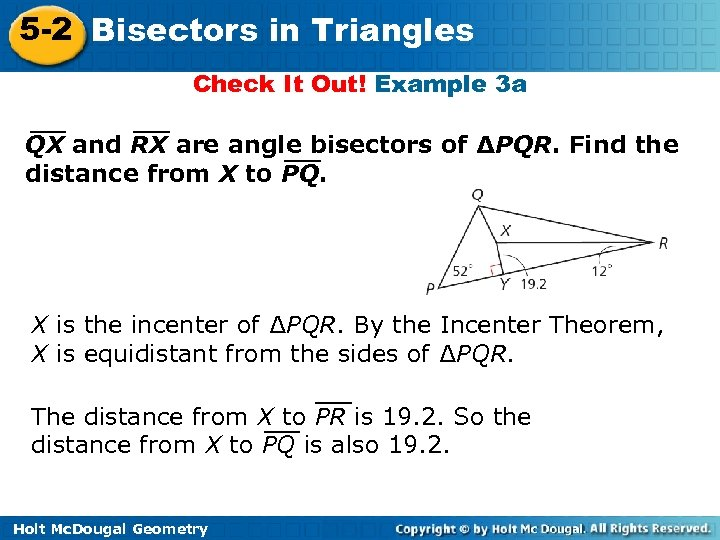5 -2 Bisectors in Triangles Check It Out! Example 3 a QX and RX are angle bisectors of ΔPQR. Find the distance from X to PQ. X is the incenter of ∆PQR. By the Incenter Theorem, X is equidistant from the sides of ∆PQR. The distance from X to PR is 19. 2. So the distance from X to PQ is also 19. 2. Holt Mc. Dougal Geometry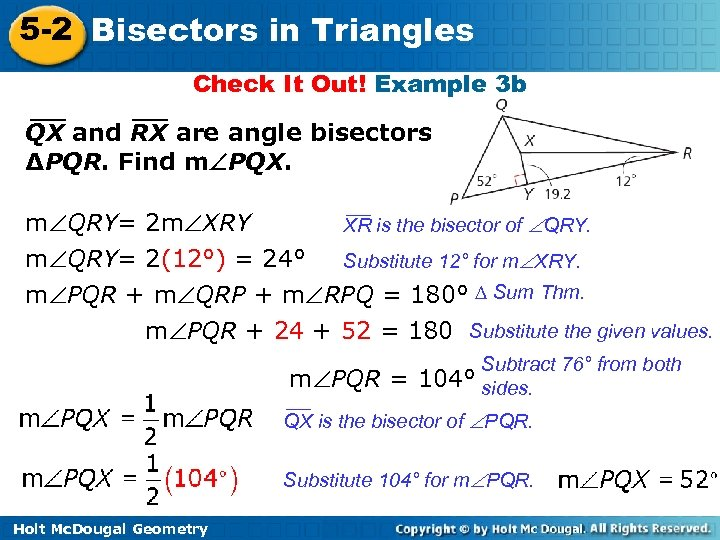5 -2 Bisectors in Triangles Check It Out! Example 3 b QX and RX are angle bisectors of ∆PQR. Find m PQX. m QRY= 2 m XRY XR is the bisector of QRY. m QRY= 2(12°) = 24° Substitute 12° for m XRY. m PQR + m QRP + m RPQ = 180° ∆ Sum Thm. m PQR + 24 + 52 = 180 Substitute the given values. m PQR = Subtract 76° from both 104° sides. QX is the bisector of PQR. Substitute 104° for m PQR. Holt Mc. Dougal Geometry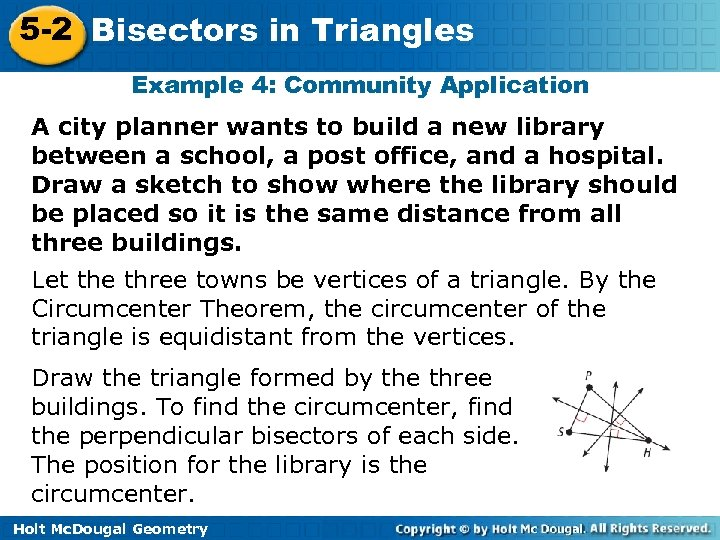5 -2 Bisectors in Triangles Example 4: Community Application A city planner wants to build a new library between a school, a post office, and a hospital. Draw a sketch to show where the library should be placed so it is the same distance from all three buildings. Let the three towns be vertices of a triangle. By the Circumcenter Theorem, the circumcenter of the triangle is equidistant from the vertices. Draw the triangle formed by the three buildings. To find the circumcenter, find the perpendicular bisectors of each side. The position for the library is the circumcenter. Holt Mc. Dougal Geometry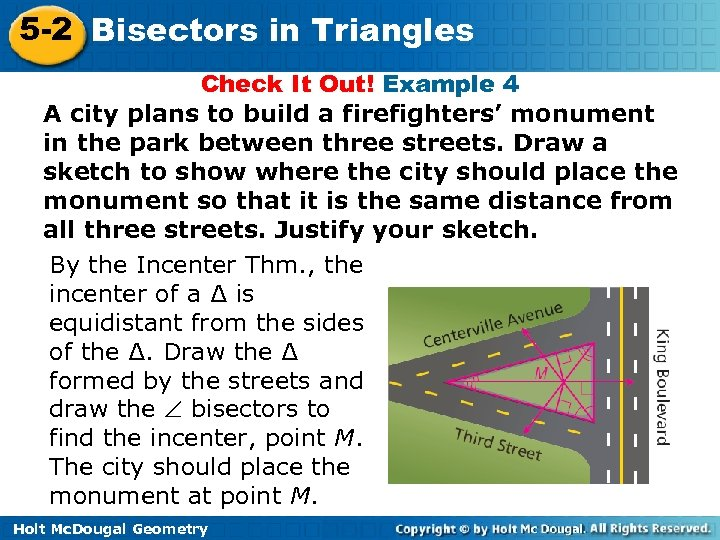5 -2 Bisectors in Triangles Check It Out! Example 4 A city plans to build a firefighters’ monument in the park between three streets. Draw a sketch to show where the city should place the monument so that it is the same distance from all three streets. Justify your sketch. By the Incenter Thm. , the incenter of a ∆ is equidistant from the sides of the ∆. Draw the ∆ formed by the streets and draw the bisectors to find the incenter, point M. The city should place the monument at point M. Holt Mc. Dougal Geometry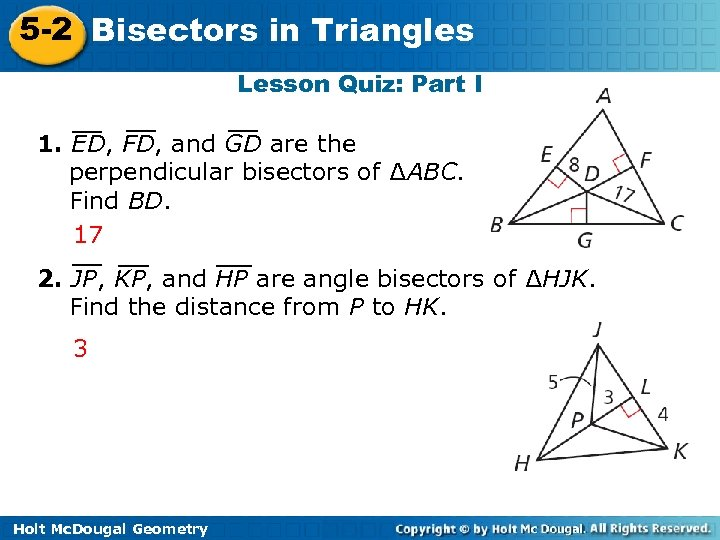5 -2 Bisectors in Triangles Lesson Quiz: Part I 1. ED, FD, and GD are the perpendicular bisectors of ∆ABC. Find BD. 17 2. JP, KP, and HP are angle bisectors of ∆HJK. Find the distance from P to HK. 3 Holt Mc. Dougal Geometry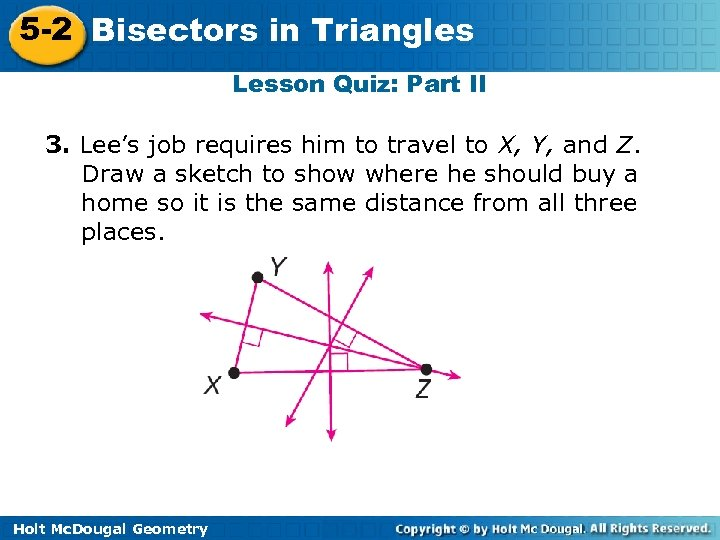5 -2 Bisectors in Triangles Lesson Quiz: Part II 3. Lee’s job requires him to travel to X, Y, and Z. Draw a sketch to show where he should buy a home so it is the same distance from all three places. Holt Mc. Dougal Geometry